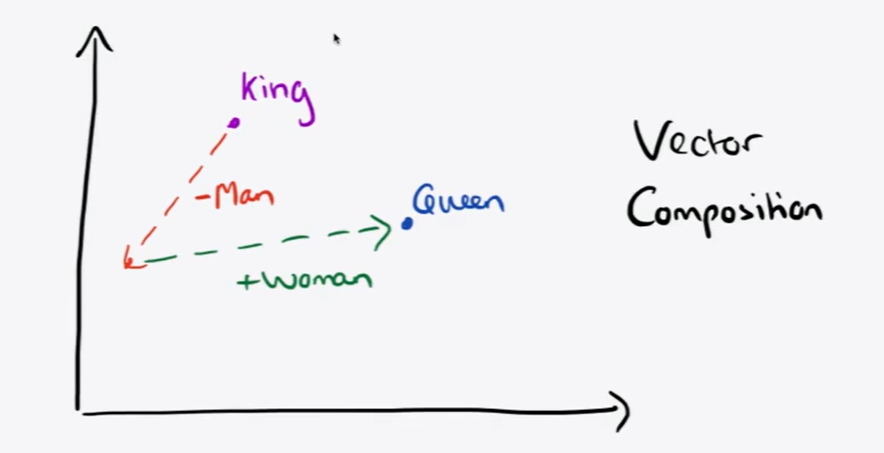# Course 2 - Word Vectors and Word Senses

Firstly, some analogy by calculating the linear space similarity by word vectors.Definition of Code:

Some Implementations:

Next thing: PCA Scatterplot

## Word Vectors and word2vec

### 1. Review: Main idea of word2vec

• Iterate through each word of the whole corpus
• Predict surrounding words using word vectors

## Optimization Basics: Gradient Descent(cs229 Optimization)

Idea: for current value of theta, calculate gradient of J(theta), then take small step in the direction of negative gradient.

Update equation(in matrix notation)

Update equation(in a single parameter)

Algorithm:

Solution: Repeatedly sample windows, and update after each one

Algorithm

### Stochastic Gradient with word vectors:

Iteratively take gradients at each such window for SGD

But in each window, we only have at most 2m+1 words, so it’s very sparse!

Solution:

• need sparse matrix update operations to only update certain rows of full embedding matrices U and V
• need to keep around a hash for word vectors

### Word2Vec: More details

Two model variants:

• Skip-grams(SG): Predict context words given center word
• Continuous Bag of Words(CBOW): Predict center word from context words

=> Skip-gram model

• Negative sampling

Paper: “Distributed Representations of Words and Phrases and their Compositionality”(Mikolov et al.2013)

Overall objective function(maximize):

The sigmoid function:

So we maximize the probability of two words co-occurring in the first log

Maximize probability that real outside word appears, minimize prob. that random word appear around center word.

The unigram distribution U(w)

raised to the 3/4 power, which makes less frequent words be sampled more often.

## Can we capture this essence more effectively by counting?

With a co-occurrence matrix X

• 2 options: windows vs. full document
• Window: Similar to word2vec, use window around each word -> captures both syntactic(POS) and semantic information
• Word-document co-occurrence matrix will give general topic leading to Latent Semantic Analysis

Models are less robust due to increase in size with vocabulary.

Solution: Low dimensional vectors

Idea: store most information in a fixed, small number of dimensions

### Hacks to X (several used in Rohde et al. 2005)

Problem: function words(the, he, has) are too frequent -> syntax has too much impact

Solution

• min(X,t) t~= 100, Ignore them all
• Use Pearson correlations instead of counts, then set negative values to 0

## The GloVe model of word vectors

### Crucial insight

Ratios of co-occurrence probabilities can encode meaning components

Merits

• Fast training
• Scalable to hug corpora
• Good performance even with small corpus and small vectors

## Evaluating word vectors

### Intrinsic

• Evaluation on a specific/intermediate subtask
• Fast to compute
• Helps to understand that system

Methods:

1. Cosine distance of word vectors
2. Word vector distances and their correlation with human judgments

### Extrinsic

• Evaluation on a real task
• Can take a long time to compute accuracy
• Unclear if subsystem is the problem or its interaction
• If replacing exactly one subsystem with another improves accuracy

Example: NER

## Word senses

#### Word senses and word sense ambiguity

Most words have lots of meanings, especially common words.